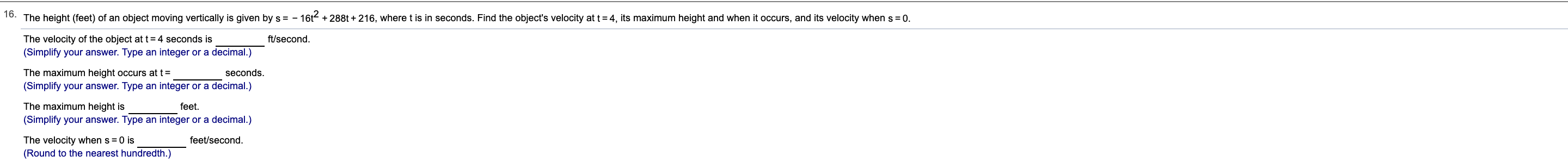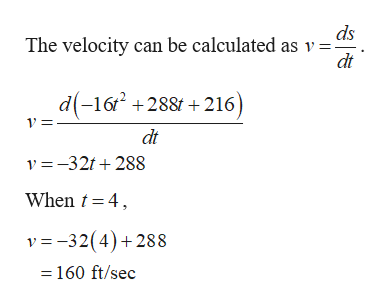# o. The height (feet) of an object moving vertically is given by s = -16t288t+216, where t is in seconds. Find the object's velocity at t 4, its maximum height and when it occurs, and its velocity when s0.The velocity of the object at t4 seconds is(Simplify your answer. Type an integer or a decimal.)ft/secondThe maximum height occurs at t=seconds.(Simplify your answer. Type an integer or a decimal.)The maximum height isfeet(Simplify your answer. Type an integer or a decimal.)feet/secondThe velocity when s 0 is(Round to the nearest hundredth.)

Question
20 views

Can I get help with this problem step by step?help_outlineImage Transcriptioncloseo. The height (feet) of an object moving vertically is given by s = - 16t288t+216, where t is in seconds. Find the object's velocity at t 4, its maximum height and when it occurs, and its velocity when s 0. The velocity of the object at t4 seconds is (Simplify your answer. Type an integer or a decimal.) ft/second The maximum height occurs at t= seconds. (Simplify your answer. Type an integer or a decimal.) The maximum height is feet (Simplify your answer. Type an integer or a decimal.) feet/second The velocity when s 0 is (Round to the nearest hundredth.) fullscreen
check_circle

Step 1

Given:

Step 2

Find the velocity when t = 4.help_outlineImage Transcriptioncloseds The velocity can be calculated as v = dt d(-16+288+216 dt v-32t288 When t 4 v -32(4)288 160 ft/sec fullscreen
Step 3

At maximum height vel...

### Want to see the full answer?

See Solution

#### Want to see this answer and more?

Solutions are written by subject experts who are available 24/7. Questions are typically answered within 1 hour.*

See Solution
*Response times may vary by subject and question.
Tagged in

### Other